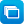# Mathematics / Year 10 / Number and Algebra / Linear and non-linear relationships

Curriculum content descriptions

Solve simple exponential equations (ACMNA270)

Elaborations
• investigating exponential equations derived from authentic mathematical models based on population growth
General capabilities
• Numeracy Numeracy
• Critical and creative thinking Critical and creative thinking
ScOT terms

Exponential functions,  Equations

## Refine results by

Year level 9-10
Resource type
Learning area Mathematics

## Refine by topic

Related topic### Using exponents in real-life contexts

This animated presentation provides four examples of the use of exponential functions to model real-life practical situations. The examples highlight the manipulation of indices (exponents) and the index laws.### Maths of mouse plagues

How do pests such as mice reach plague proportions? The answer is that they can grow at an exponential rate. Watch this clip to see how that happens, but first enjoy (or squirm at) some footage of a real mouse plague.### Exponential growth and doubling time

This resource aims to guide and support a mathematics teacher of Year 10 across three main areas as the class explores the mathematics of exponential growth. The contexts used are compound interest, animal and human population growth, and the growth (and decay) of bacteria populations.### Calculations involving exponential growth: world population

Recently the world's population passed seven billion and it continues to grow by 200,000 people every day. Listen to some reasons why our population is growing at such a rate, and the strain this will put on resources such as food and water. This clip provides context for calculations involving exponential growth.### Linear equations and inequalities

This resource is a collection of screen displays of questions relating to linear equations and inequations. Graphing tasks involve determining the coordinates of two points on the linear function. Other tasks are presented as multiple-choice questions and involve finding slopes of lines, calculating intercepts with the ...### Secondary mathematics: different representations

These seven learning activities, which focus on 'representations' using a variety of tools (software) and devices (hardware), illustrate the ways in which content, pedagogy and technology can be successfully and effectively integrated in order to promote learning. In the activities, teachers use different representations ...### TIMES Module 8: Statistics and Probability: data investigation and interpretation, year 10 - teacher guide

This is a 29-page guide for teachers. Box plots are introduced as another graphical tool and are used for comparisons of data. Scatter plots are used to explore relationships between quantitative (usually continuous) variables.### Maths and the car: loan calculator

Use a calculator to estimate loan repayments needed to buy a car. Look at variables such as price, compound interest rate, term of loan and payment frequency. Work out the total interest to be paid by the borrower.### TIMES Module 22: Number and Algebra: consumer arithmetic - teacher guide

This is a 23-page guide for teachers, providing an introduction to the financial mathematics component of the number and algebra strands for years 9 and 10. A brief history of the concept of money concludes the module.### TIMES Module 33: Number and Algebra: factorisation - teacher guide

This is a 17-page guide for teachers. It continues the discussion of factorisation. In particular, the techniques for the factorisation of quadratic expressions are presented.### Laptop wrap: Parallelendicular

Students construct a series of GeoGebra applets that investigate the parameters gradient and intercepts of straight lines. They reinforce this knowledge with Microsoft Math 3.0.### Simultaneous Equations

A short introduction to simultaneous equations and how they are solved.### Simultaneous Equations: animation

A short animated introduction to simultaneous equations and a demonstration of the elimination method of solution, followed by an interactive quiz.### TIMES Module 36: Number and Algebra: formulas - teacher guide

This is a 13-page guide for teachers. It introduces the use of formulas, and includes substitution and the solution of the resulting equations.### reSolve: Real World Algebra: Quadratic Functions

This sequence of three lessons explores different representations of quadratic functions, with particular emphasis on turning points in quadratic graphs. Students use images of trajectories, inscribe their own curves using Geogebra and then further explore the algebraic representation of their curves. Each lesson is outlined ...### Introduction to differential calculus

This collection of 17 digital curriculum resources (all learning objects) is organised into three sections: rates of change; differential calculus; and review of differential calculus via three learning objects. Students explore rates of change through liquid-pouring activities, and are introduced to the gradient of secants ...### Graphing functions

This collection contains five digital curriculum resources (all interactive learning objects) that introduce students to graphing linear, parabolic, cubic and trigonometric functions. Through exercises, students investigate how the constants in each function equation influence relevant aspects of graphing, such as dilations ...### Non-linear systems of equations

This digital resource presents a video demonstration, with commentary, of the pen-and-paper technique of constructing the graphs of linear and quadratic functions to find the solutions to a non-linear system of equations. In a single example, a step-by-step illustration of the written method is presented, complemented by ...### S-tunes

Are music downloads a CD swan song? The application form for a radio DJ included the question 'What CD do you currently have loaded in your CD player?' The young applicant responded, 'I don’t own a CD player.' The Y-gen are techno savvy, but at what cost? A song from popular online stores costs around \$1.30 each. A compilation ...### reSolve: Real World Algebra: Exponential Functions

This sequence of two lessons explores exponential functions as seen in the real world, linking the representations of function rules, graphs and tables of values. Students consider real world objects and data that can be described using exponential functions. They use simulations and modelling to investigate transformations, ...